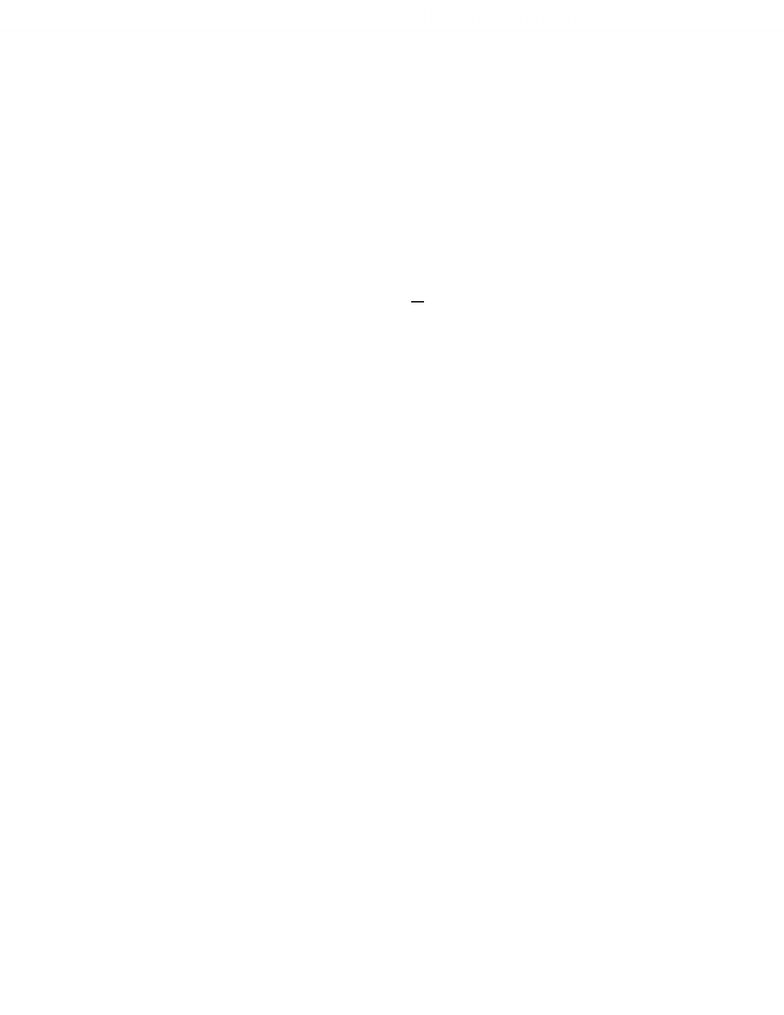Study Guides (390,000)
CA (150,000)
UTSG (10,000)
MAT (1,000)
all (20)
Midterm

# MAT136H1 Study Guide - Midterm Guide: Improper Integral

Department
Mathematics
Course Code
MAT136H1
Professor
all
Study Guide
Midterm

This preview shows half of the first page. to view the full 1 pages of the document.11.4 Infinite Sequences & Series
Comparison Tests: Overview
The Comparison Test
If and are series with positive terms
1) If is convergent and   for all , then is also convergent
2) If is divergent and   for all , then is also divergent
Limit Comparison Test
For series with positive terms and , if 
  for a finite number  , then both
series converge or both series diverge.
Estimating Sums
Based on the first number of terms in the series, the sum can be estimated. The error is than what
remains from the next term thereafter until infinity. This is calculated by     
       and its lower and upper limit can be set using improper integral:

  